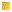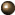Sensors & Transducers Journal     (ISSN: 2306-8515, e-ISSN 1726-5479)

 About this Journal    Information for Authors    Browse Journal Submit Press Release Submit White Paper 25 Top Downloaded Articles (2007-2012)

Vol. 226, Issue 10, October 2018, pp. 37-46Embedding the Photon with Its Relativistic Mass as a Particle into the Electromagnetic Wave Explains the Gouy Phase Shift as an Energetic Effect Konrad ALTMANN LAS-CAD GmbH, Brunhildenstrasse 9, 80639 Munich, GermanyTel.: + 49 80 173607, fax: + 49 89 172594 * E-mail: dr.altmann@las-cad.com   Received: 25 June 2018 /Accepted: 31 August 2018 /Published: 31 October 2018

Abstract: A new aspect concerning the relationship between photon and electromagnetic wave has been developed by considering the question why the energy and the mass density of an electromagnetic wave are propagating in the same direction. For instance, in optical resonators the energy density usually propagates along curved lines. However, according to Newton's first law the mass density should propagate along a straight line, if no force is exerted it. In order to solve this problem, the assumption has been made that a transverse force is exerted on the mass density and in consequence on the mass of the photons which forces them to follow the propagating energy density. The expression obtained for this force makes it possible to show that the photon is moving within a transverse potential. This finally leads to the result that the transverse probability density of the photons can be computed by solving a Schrödinger equation identical with the Schrödinger equation describing the motion of the electron, except that the mass of the electron is replaced by the relativistic mass of the photon. In this way, it could for the first time be shown that the Schrödinger equation is also describing the motion of a particle which has no rest mass. The results obtained in this way for the intensity distribution of Gaussian waves and the Gouy phase shift are in full agreement with the results obtained by the use of wave optics. In addition, the Gouy phase shift could be explained as an energetic effect. For this purpose, it has been shown that the energy, which is obtained by multiplying the effective axial propagation constant by kz(z), is equal to the difference between the total energy Eph of the photon and the energy eigenvalues Enm(z) of the Schrödinger equation.

Keywords: Quantum optics, Electromagnetic wave, Paraxial wave optics, Physical optics, Laser theory, Laser resonators.Click <here> or title of paper to download the full pages article in pdf format